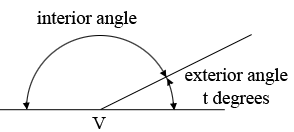SEARCH HOMEMath Central Quandaries & QueriesQuestion from percy, a student: a regular polygon has n sides .The size of each interior angle is eight times the size of each exterior angle . 1.find the size of each exterior angle 2.calculate the value of nHi Percy,

Below is a diagram of one of the vertices, $V$ of the polygon with the interior and exterior labeled.The measure of the exterior angle is $t$ degrees. What is the measure of the interior angle in terms of $t?$ The measure of the interior angle is 8 times the measure of the exterior angle. Solve for $t.$

For part 2 I suggest you look at Walters response to Evelina.

PennyMath Central is supported by the University of Regina and the Imperial Oil Foundation.